# formula for cylinder

## Mensuration of Class 8

A cylinder is a three dimension figure .its two bases are circles and the sides of a cylinder are curve.

 Solids like measuring jars, circular pillars, circular pencils, circular pipes, road rollers, gas cylinders etc., are said to have cylindrical shape. For a cylinder of base radius = r and height (or length) = h, we have Volume = (πr2h) cubic units Curved surface area = 2πrhsq units Total surface area = (2πrh + 2πr2) sq units           = 2πr(h + r) sq units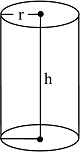### HOLLOW CYLINDERS

Solids like iron pipes, rubber tubes are in the shape of hollow cylinders.

Consider a hollow cylinder having

External radius = R, internal radius = r and height = h.

Then, we have

• Volume of material = (external volume) – (internal volume)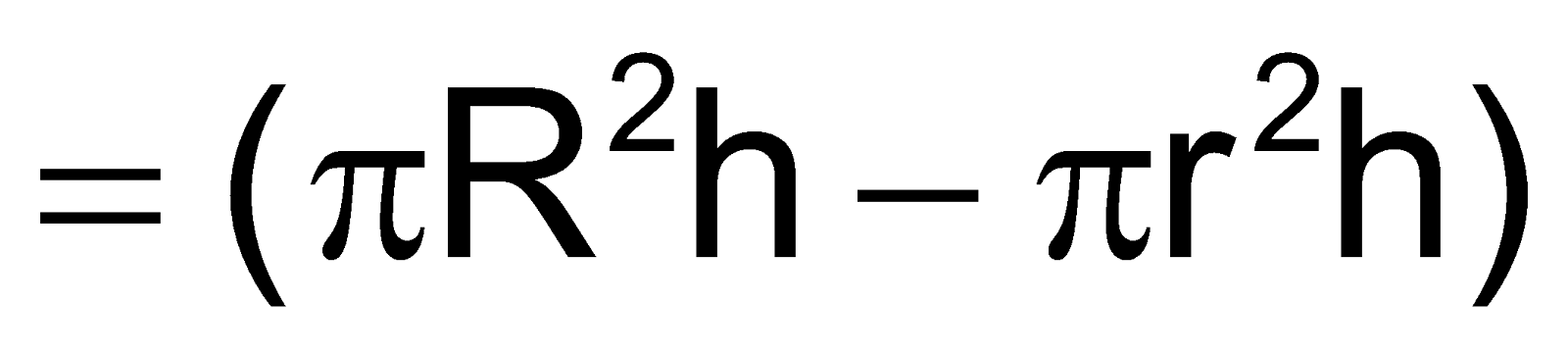cubic units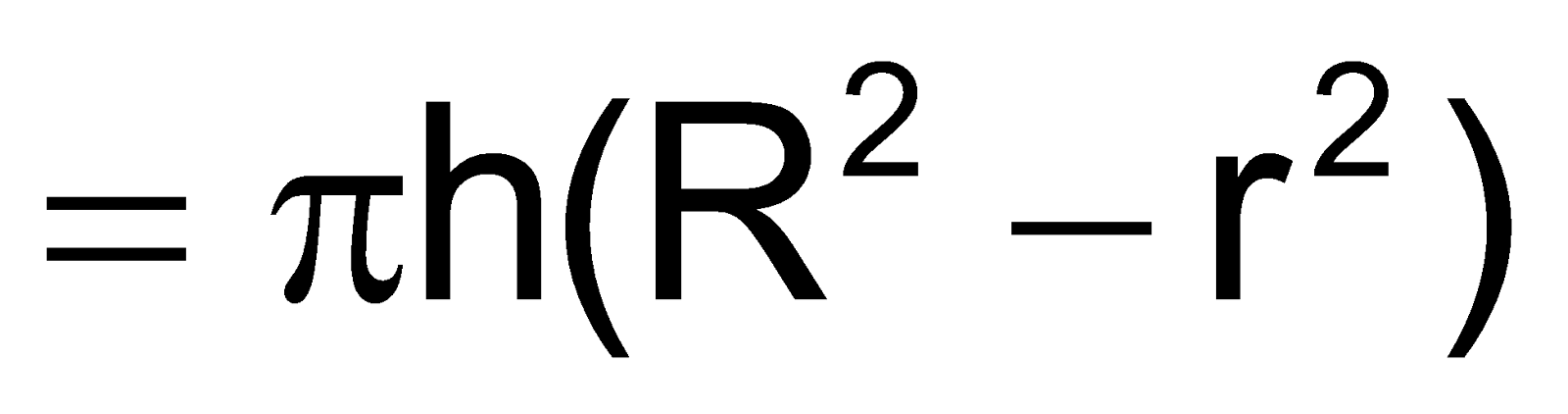cubic units

• Curved surface area of hollow cylinder

= (external surface area) – (internal surface area)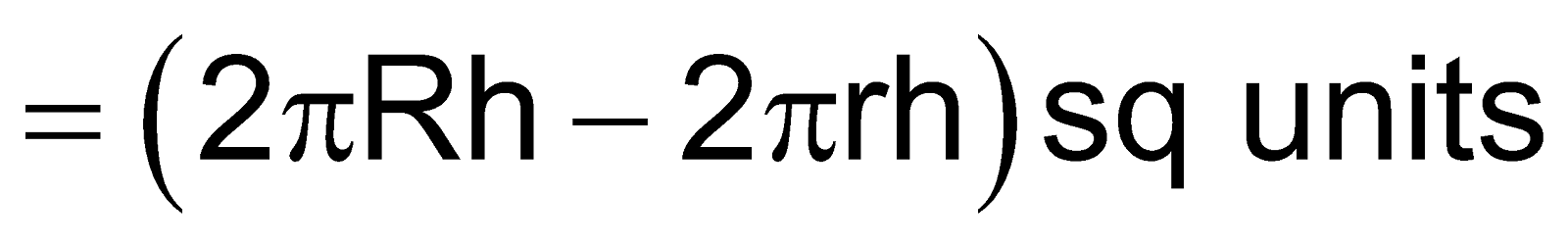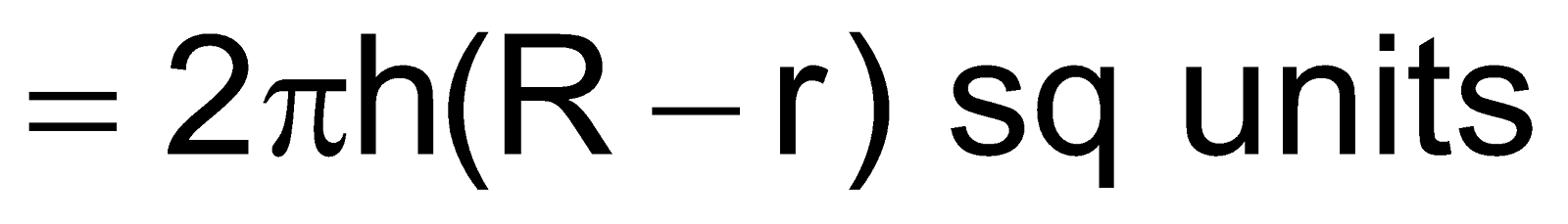• Total surface area of hollow cylinder

=(curved surface area) + (area of the base ring)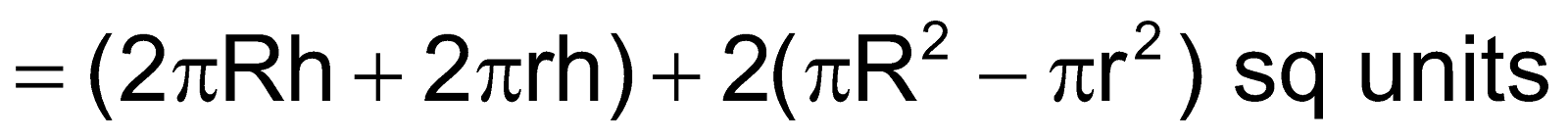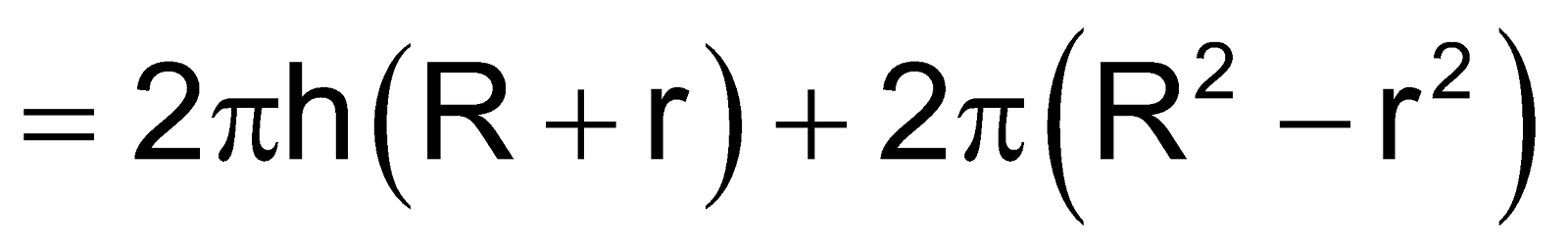sq units

### SOLVED EXAMPLES

question Find the volume of cylinder if the radius of a cyinder is 5m and its height is 10m.

Sol.   Here, h = 10 m and r = 5 m.

So, volume of cylinder is = πr2h

=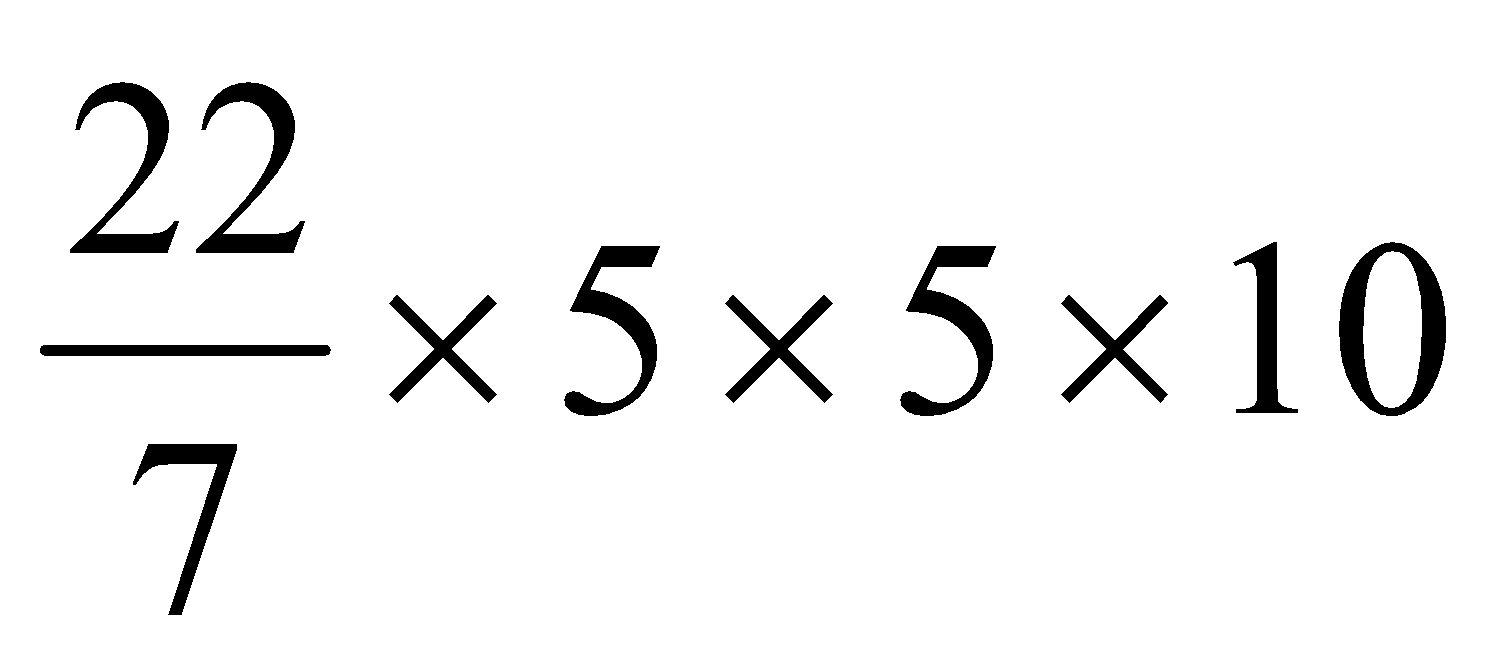= 785.71 m3

question A closed cylindrical tank of radius 8 m and height 15 m is made from a sheet of metal. How much sheet of metal is required?

Sol.Total surface area of cylinder = 2πr (r + h)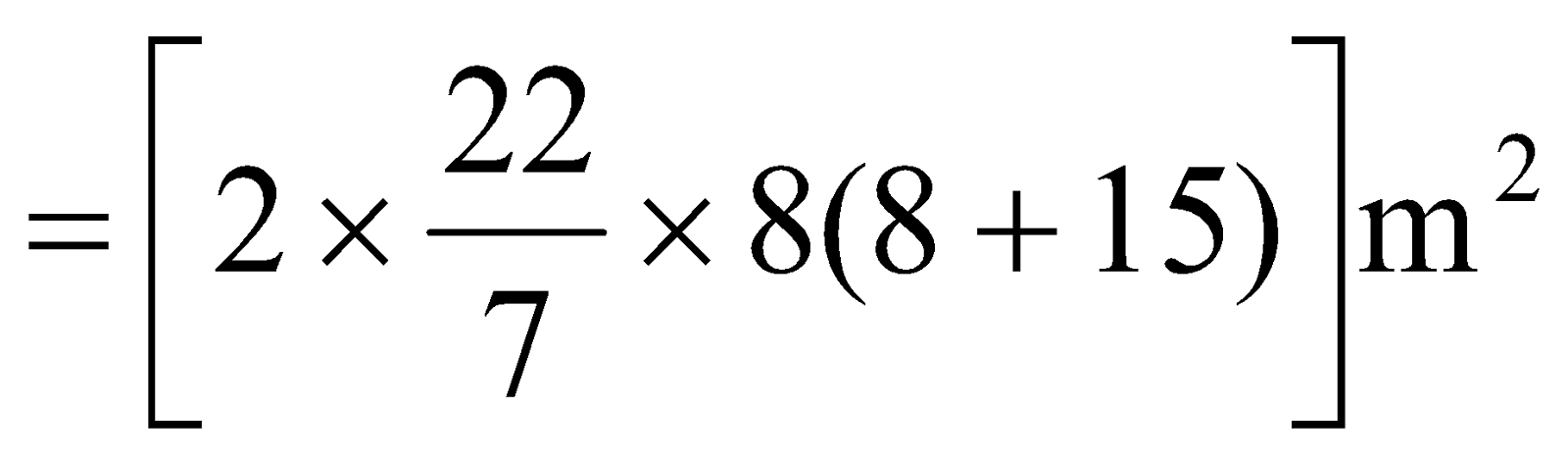= 1156.57 m2

Thus, 1156.57 m2 sheet of metal is required.

## CBSE NCERT Solutions for Class 8 Maths

Notes,worksheet and solved question for Maths class 8

Check your marks in a chapter which you have complited in school from Physics Wallah chapter wise online test just click on the link given below